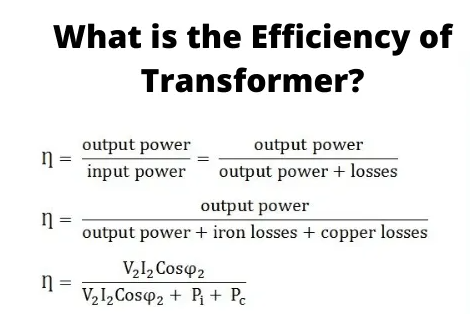# EFFICIENCY OF TRANSFORMER

## Introduction of Efficiency of Transformer

Transformers create the most important connection between load and supply systems. The transformer’s efficiency has a direct effect on its performance and aging. In general, the efficiency of a transformer is in the range of 95 – 99 %. The efficiency of large power transformers with very low losses can be as high as 99.7%. The output and input of a transformer are not measured under loaded conditions when the wattmeter readings inevitably suffer errors of 1 – 2%. So Using OC and SC tests is to calculate rated core and winding losses in the transformer for the purpose of efficiency calculations. The copper losses depend on the currents through the transformer primary and secondary windings and the core losses depend on the transformer rated voltage. Therefore, transformer efficiency plays an important role in operating it under constant voltage and frequency conditions. The temperature rise of the transformer due to heat generated has an effect on the life of transformer oil properties and decides the reasonable type of cooling method. The rating of the equipment is limited by the temperature rise. The transformer’ efficiency  is simply shown as:• The output power is the result of the fraction of power factor of the load and the rated loading (volt-ampere).
• The losses are the sum of copper losses in the windings + the iron loss + stray load loss +dielectric loss.
• The iron losses include the eddy and hysteresis current losses in the transformer. These losses depend on the flux density inside the core. Mathematically,• kh and ke are constants
• Bmax is the peak magnetic field density
• f is the source frequency
• t is the thickness of the core.
• The power ‘n’ in the hysteresis loss is known as the Steinmetz constant whose value can be nearly 2.• The dielectric losses occur inside the transformer oil. For low voltage transformers, it can be neglected.
• The leakage flux connects to the metal frame, tank, etc. to produce eddy currents and are present all around the transformer hence called stray loss, and it depends on the load current and is so named as ‘stray load loss.’ It can be represented by resistance in series to the leakage reactance.

## Calculating Efficiency of the Transformer

The equivalent circuit of the transformer referred to the primary side is given below. Here Rc accounts for core losses. Using the Short circuit (SC) test, we can find the equivalent resistance accounting for copper losses as:• x%: the percentage of full or rated load ‘S’ (VA)
• Pcufl (watts): the full load copper loss and cosθ be the power factor of the load.
• Pi (watts): core loss.

As copper and iron losses are major losses in the transformer hence only these two types of losses are taken into account while calculating efficiency. Then the efficiency of the transformer can be written as :Where,

The maximum efficiency (ηmax) occurs when the variable losses are equal to the constant losses. Since the copper loss is load-dependent, hence it is a variable loss quantity. And the core loss is taken to be the constant quantity. So the condition for maximum efficiency is:Now maximum efficiency is written as :This indicated that it is possible to obtain maximum efficiency at full load by proper selection of constant and variable losses. However, it is difficult to obtain maximum efficiency as the fixed core losses are less lower higher than copper losses.
The variation of efficiency with loading can be shown by the figure below:It is seen that the figure that the maximum efficiency takes place at the unity power factor. And the maximum efficiency takes place at the same loading irrespective of the power factor of the load.

## All Day Efficiency of Transformer

This is an energy-based performance calculation for a distribution transformer. Unlike a power transformer which is switched on or off depending on the load, it handles; a load of a distribution transformer fluctuates continuously 24 hours a day. Since core loss is independent of load, all-day efficiency depends on copper loss. It is defined as the ratio of output energy delivered to input energy for a 24-hour cycle. High energy efficiencies are gained by limiting core flux densities to lower values (as the core losses are dependent on flux density) by using a relatively larger cross-section or larger iron/copper weight ratio.

.(+84) 0913.006.538
Skype, Telegram, Wechat ...